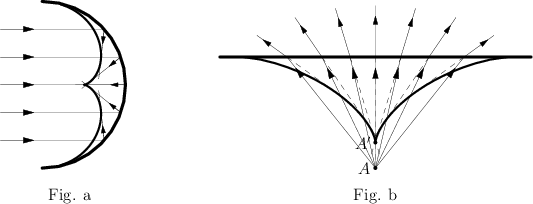# Caustic

(Redirected from Catacaustic)For example, the catacaustic of a parallel beam of rays reflected from a semi-circle is part of an epicycloid (see Fig. a); the diacaustic of a pencil of rays emanating from a point $A$ lying in a denser medium and refracted by a straight line is part of an astroid with cusp $A'$ the distance of which from the line is $1/n$ times that of the point $A$ (where $n$ is the refraction index) (see Fig. b).